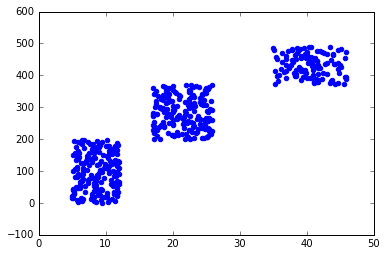• No products in the cart.

# 204.6.6 Practice : Kernel – Non Linear Classifier

##### Putting Kernels into practice.

Link to the previous post : https://statinfer.com/204-6-5-the-non-linear-decision-boundary/

In this session we will practice non linear kernels of SVM in python.

### Practice : Kernel – Non linear classifier

• Dataset : Software users/sw_user_profile.csv
• How many variables are there in software user profile data?
• Plot the active users against and check weather the relation between age and “Active” status is linear or non-linear.
• Build an SVM model(model-1), make sure that there is no kernel or the kernel is linear.
• For model-1, create the confusion matrix and find out the accuracy.
• Create a new variable. By using the polynomial kernel.
• Build an SVM model(model-2), with the new data mapped on to higher dimensions. Keep the default kernel as linear.
• For model-2, create the confusion matrix and find out the accuracy.
• Plot the SVM with results.
• With the original data re-cerate the model(model-3) and let python choose the default kernel function.
• What is the accuracy of model-3?
In :
#Dataset : Software users/sw_user_profile.csv

In :
#How many variables are there in software user profile data?
sw_user_profile.shape

Out:
(490, 3)
In :
#Plot the active users against and check weather the relation between age and "Active" status is linear or non-linear
plt.scatter(sw_user_profile.Age,sw_user_profile.Id,color='blue')

Out:
<matplotlib.collections.PathCollection at 0xce7ac50>In :
#Build an SVM model(model-1), make sure that there is no kernel or the kernel is linear

#Model Building
X= sw_user_profile[['Age']]
y= sw_user_profile[['Active']].values.ravel()
Linsvc = svm.SVC(kernel='linear', C=1).fit(X, y)

In :
#Predicting values
predict3 = Linsvc.predict(X)

In :
#For model-1, create the confusion matrix and find out the accuracy
#Confusion Matrix
from sklearn.metrics import confusion_matrix
conf_mat = confusion_matrix(sw_user_profile[['Active']],predict3)
conf_mat

Out:
array([[317,   0],
[173,   0]])
In :
#Accuracy
Accuracy3 = Linsvc.score(X, y)
Accuracy3

Out:
0.64693877551020407

New variable derivation. Mapping to higher dimensions

In :
#Standardizing the data to visualize the results clearly
sw_user_profile['age_nor']=(sw_user_profile.Age-numpy.mean(sw_user_profile.Age))/numpy.std(sw_user_profile.Age)

In :
#Create a new variable. By using the polynomial kernel
#Creating the new variable
sw_user_profile['new']=(sw_user_profile.age_nor)*(sw_user_profile.age_nor)

In :
#Build an SVM model(model-2), with the new data mapped on to higher dimensions. Keep the default kernel as linear

#Model Building with new variable
X= sw_user_profile[['Age']+['new']]
y= sw_user_profile[['Active']].values.ravel()
Linsvc = svm.SVC(kernel='linear', C=1).fit(X, y)
predict4 = Linsvc.predict(X)

In :
#For model-2, create the confusion matrix and find out the accuracy
#Confusion Matrix
conf_mat = confusion_matrix(sw_user_profile[['Active']],predict4)
conf_mat

Out:
array([[317,   0],
[  0, 173]])
In :
#Accuracy
Accuracy4 = Linsvc.score(X, y)
Accuracy4

Out:
1.0
In :
#With the original data re-cerate the model(model-3) and let python choose the default kernel function.
########Model Building with radial kernel function
X= sw_user_profile[['Age']]
y= sw_user_profile[['Active']].values.ravel()
Linsvc = svm.SVC(kernel='rbf', C=1).fit(X, y)
predict5 = Linsvc.predict(X)
conf_mat = confusion_matrix(sw_user_profile[['Active']],predict5)
conf_mat

Out:
array([[317,   0],
[  0, 173]])
In :
#Accuracy model-3
Accuracy5 = Linsvc.score(X, y)
Accuracy5

Out:
1.0

The next post is about soft margin classification noisy data.
Link to the next post : https://statinfer.com/204-6-7-soft-margin-classification-noisy-data/
21st June 2017

### 0 responses on "204.6.6 Practice : Kernel - Non Linear Classifier"

Statinfer Software Solutions LLP

Software Technology Parks of India,
NH16, Krishna Nagar, Benz Circle,